# Counting Sort

• Difficulty Level : Easy
• Last Updated : 21 Jun, 2022

Counting sort is a sorting technique based on keys between a specific range. It works by counting the number of objects having distinct key values (kind of hashing). Then do some arithmetic to calculate the position of each object in the output sequence.

### Characteristics of counting sort:

• Counting sort makes assumptions about the data, for example, it assumes that values are going to be in the range of 0 to 10 or 10 – 99 etc, Some other assumptions counting sort makes are input data will be all real numbers.
• Like other algorithms this sorting algorithm is not a comparison-based algorithm, it hashes the value in a temporary count array and uses them for sorting.
• It uses a temporary array making it a non In Place algorithm.

Let us understand it with the help of an example.

• For simplicity, consider the data in the range 0 to 9.
• Input data: {1, 4, 1, 2, 7, 5, 2}
• Take a count array to store the count of each unique object.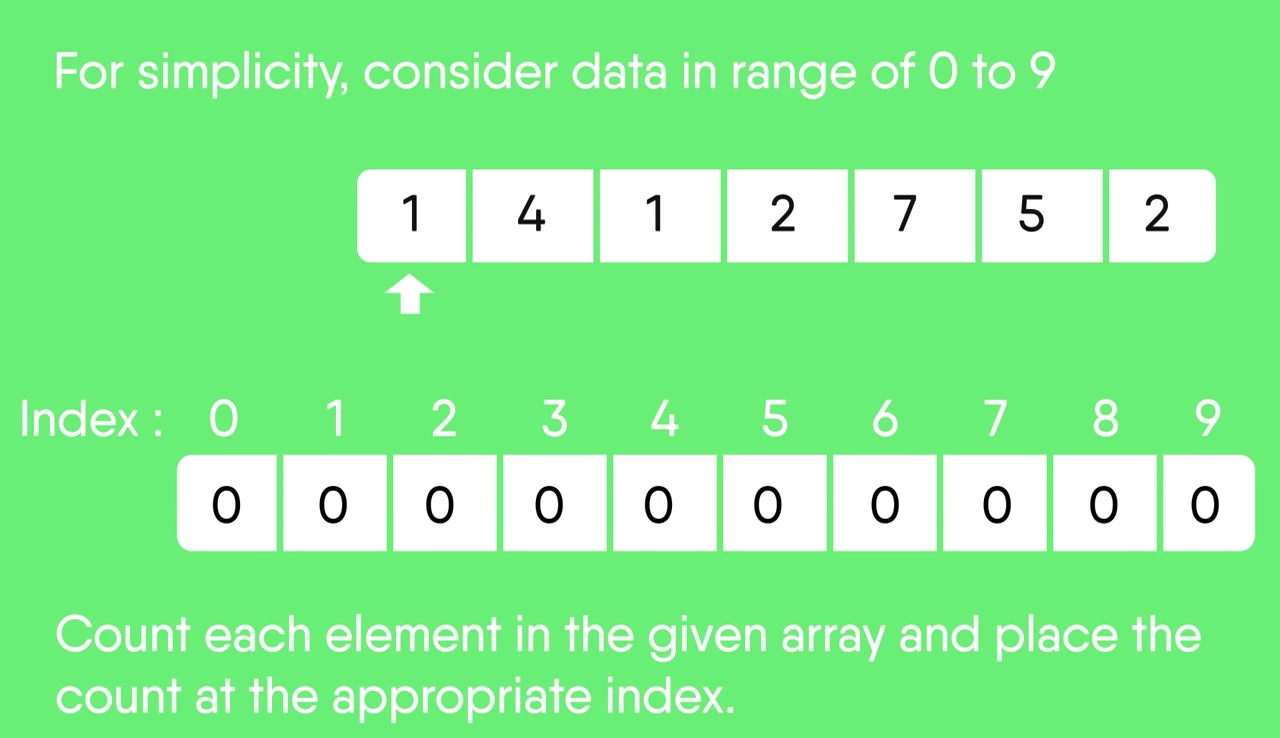• Now, store the count of each unique element in the count array
• If any element repeats itself, simply increase its count.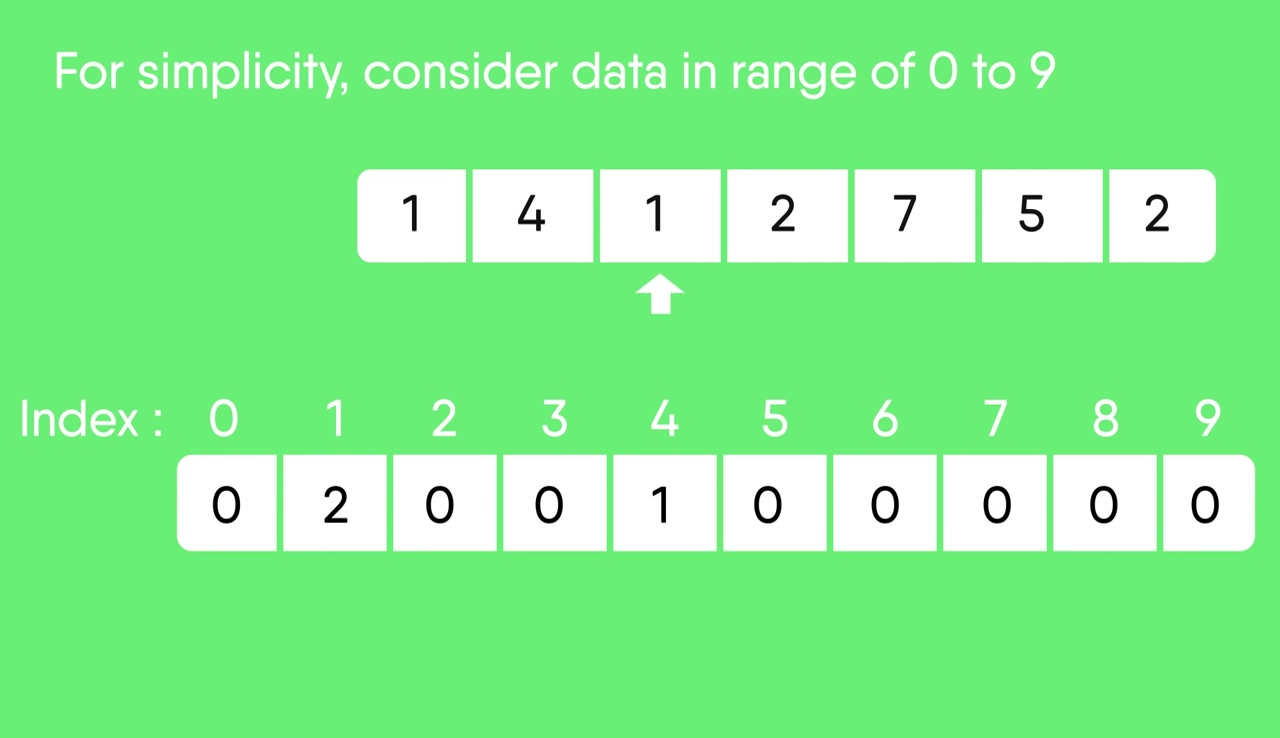• Here, the count of each unique element in the count array is as shown below:
• Index:     0  1  2  3  4  5  6  7  8  9
• Count:    0  2  2  0   1  1  0  1  0  0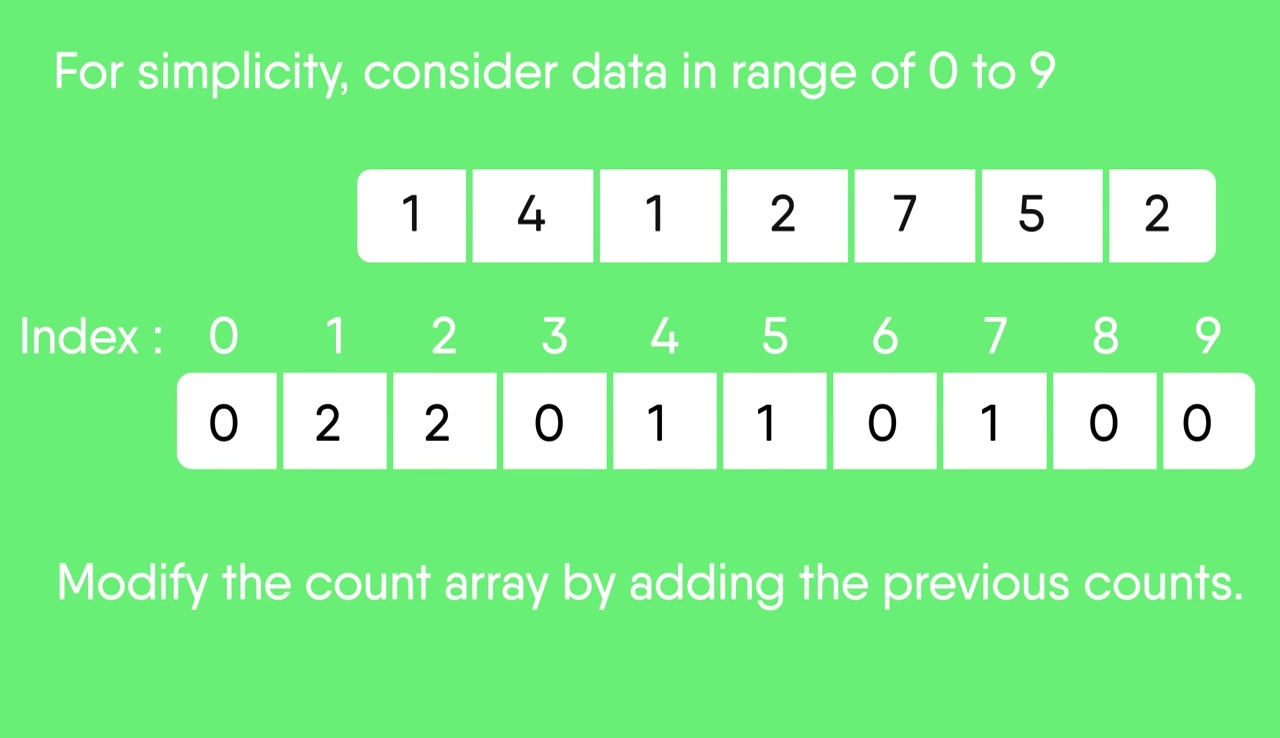• Modify the count array such that each element at each index stores the sum of previous counts.
• Index:      0  1  2  3  4  5  6  7  8  9
• Count:     0  2  4  4  5  6  6  7  7  7
• The modified count array indicates the position of each object in the output sequence.
• Find the index of each element of the original array in the count array. This gives the cumulative count.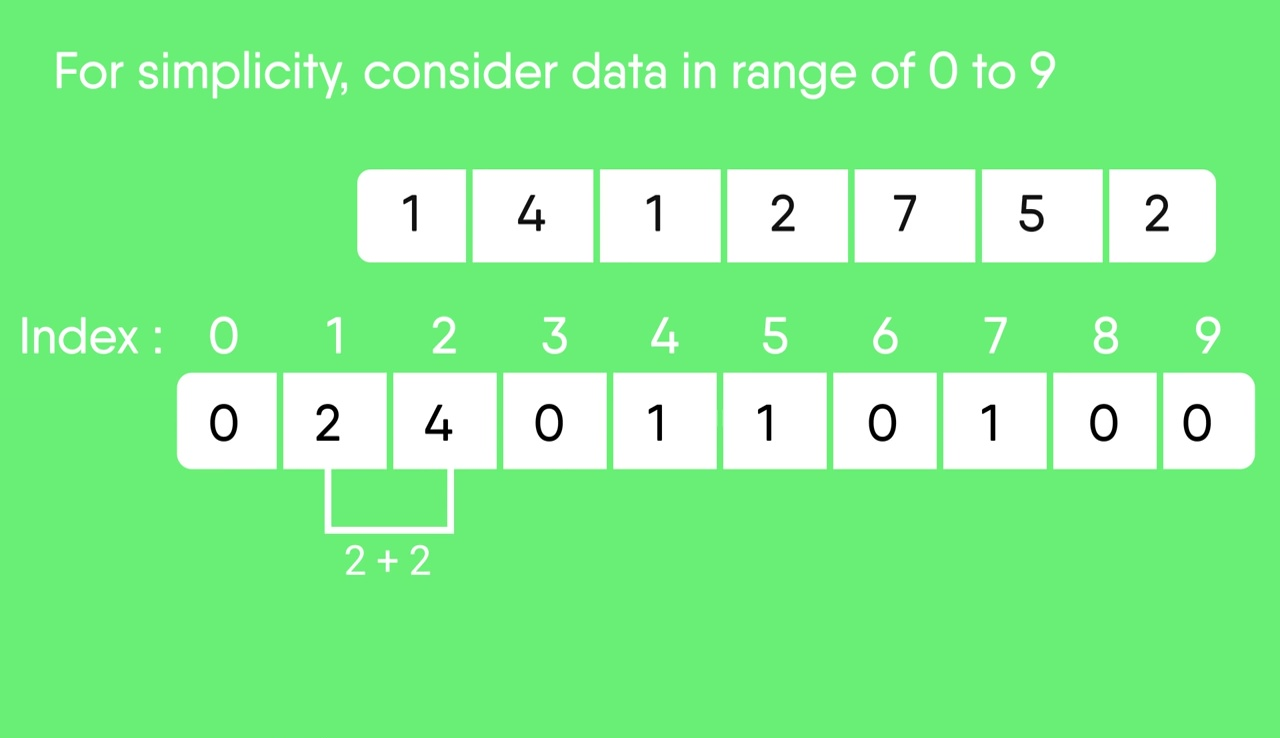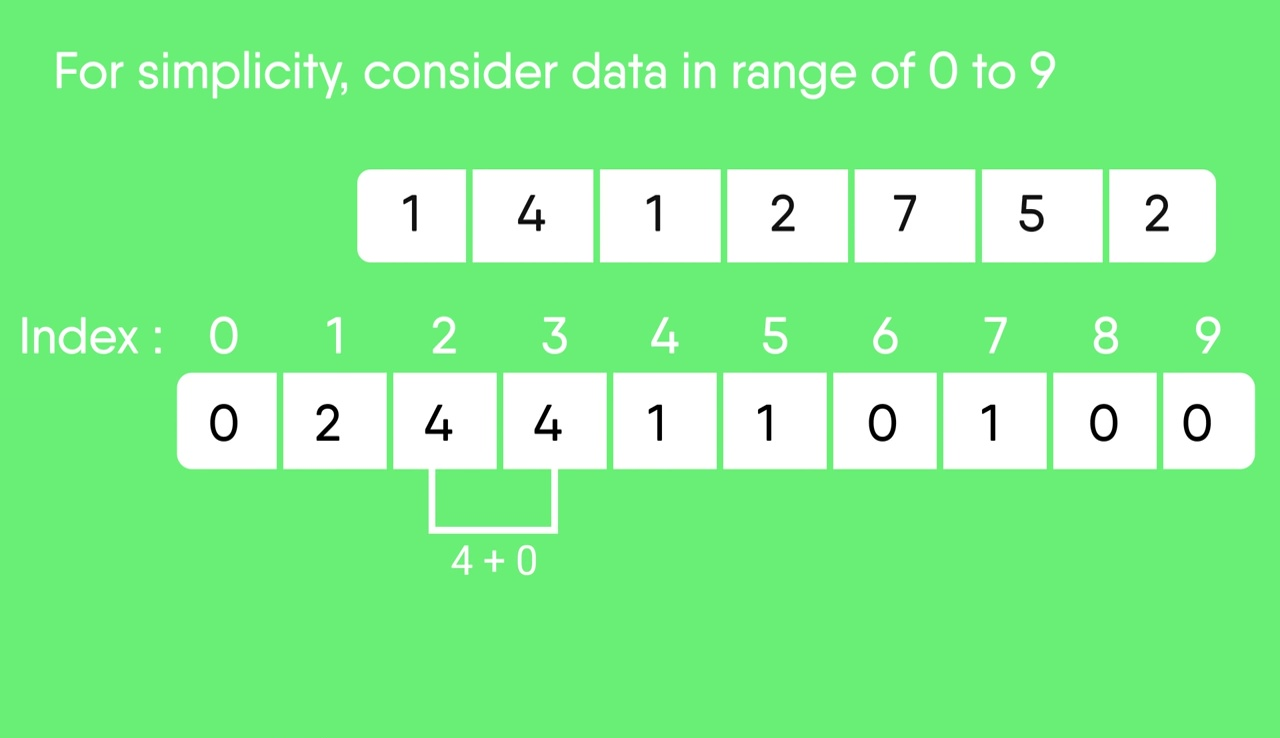• Rotate the array clockwise for one time.
• Index:     0 1 2 3 4 5 6 7 8 9
• Count:     0 0 2 4 4 5 6 6 7 7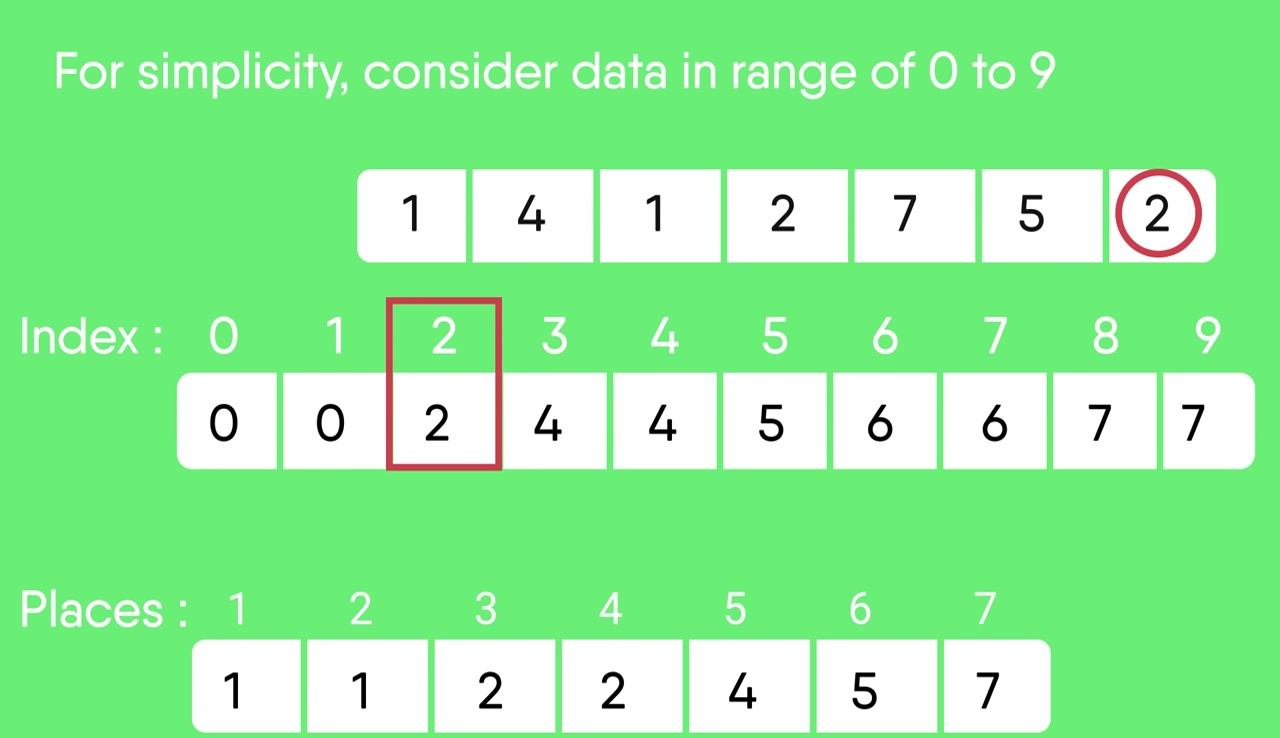•   Output each object from the input sequence followed by increasing its count by 1.
•   Process the input data: 1, 4, 1, 2, 7, 5, 2. Position of 1 is 0.
•   Put data 1 at index 0 in output. Increase count by 1 to place next data 1 at an index 1 greater than this index.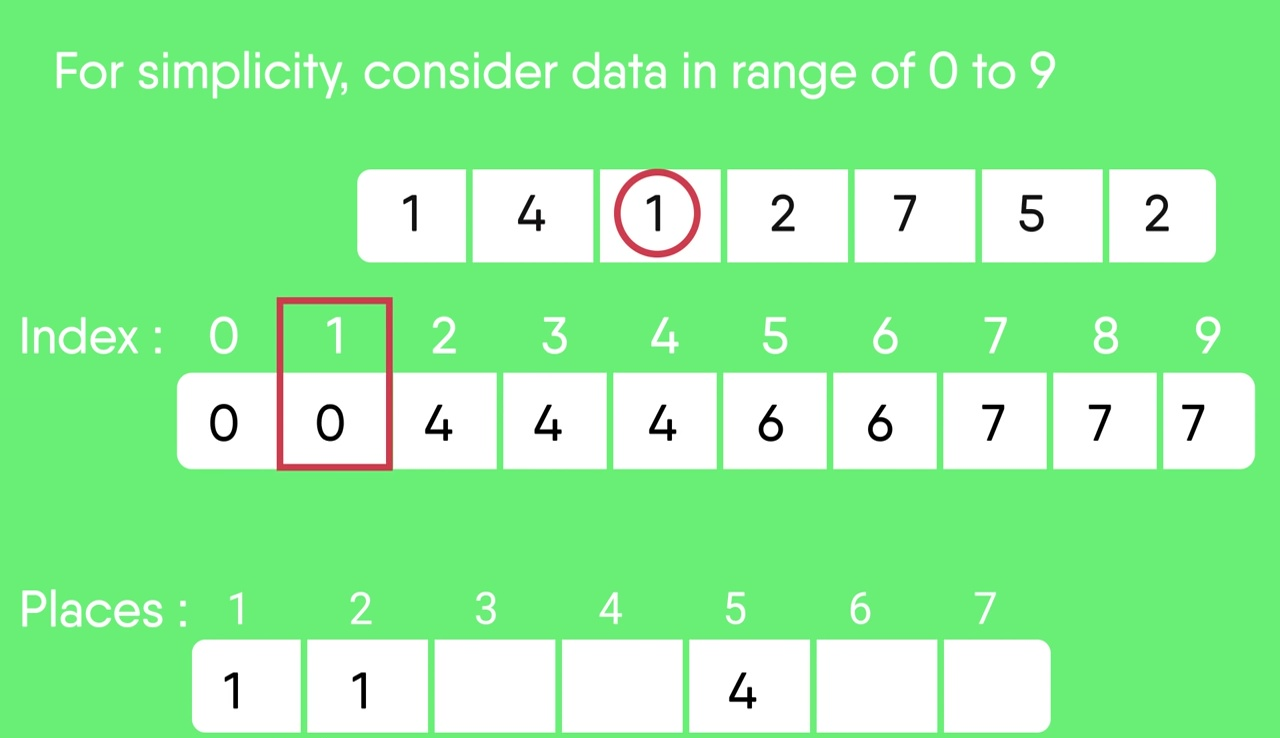• After placing each element at its correct position, decrease its count by one.
Recommended Practice

Following is the implementation of the counting sort.

## C++

 `// C++ Program for counting sort``#include ``#include ``using` `namespace` `std;``#define RANGE 255` `// The main function that sort``// the given string arr[] in``// alphabetical order``void` `countSort(``char` `arr[])``{``    ``// The output character array``    ``// that will have sorted arr``    ``char` `output[``strlen``(arr)];` `    ``// Create a count array to store count of individual``    ``// characters and initialize count array as 0``    ``int` `count[RANGE + 1], i;``    ``memset``(count, 0, ``sizeof``(count));` `    ``// Store count of each character``    ``for` `(i = 0; arr[i]; ++i)``        ``++count[arr[i]];` `    ``// Change count[i] so that count[i] now contains actual``    ``// position of this character in output array``    ``for` `(i = 1; i <= RANGE; ++i)``        ``count[i] += count[i - 1];` `    ``// Build the output character array``    ``for` `(i = 0; arr[i]; ++i) {``        ``output[count[arr[i]] - 1] = arr[i];``        ``--count[arr[i]];``    ``}` `    ``/*``    ``For Stable algorithm``    ``for (i = sizeof(arr)-1; i>=0; --i)``    ``{``        ``output[count[arr[i]]-1] = arr[i];``        ``--count[arr[i]];``    ``}``    ` `    ``For Logic : See implementation``    ``*/` `    ``// Copy the output array to arr, so that arr now``    ``// contains sorted characters``    ``for` `(i = 0; arr[i]; ++i)``        ``arr[i] = output[i];``}` `// Driver  code``int` `main()``{``    ``char` `arr[] = ``"geeksforgeeks"``;` `    ``countSort(arr);` `    ``cout << ``"Sorted character array is "` `<< arr;``    ``return` `0;``}` `// This code is contributed by rathbhupendra`

## C

 `// C Program for counting sort``#include ``#include ``#define RANGE 255` `// The main function that sort the given string arr[] in``// alphabetical order``void` `countSort(``char` `arr[])``{``    ``// The output character array that will have sorted arr``    ``char` `output[``strlen``(arr)];` `    ``// Create a count array to store count of individual``    ``// characters and initialize count array as 0``    ``int` `count[RANGE + 1], i;``    ``memset``(count, 0, ``sizeof``(count));` `    ``// Store count of each character``    ``for` `(i = 0; arr[i]; ++i)``        ``++count[arr[i]];` `    ``// Change count[i] so that count[i] now contains actual``    ``// position of this character in output array``    ``for` `(i = 1; i <= RANGE; ++i)``        ``count[i] += count[i - 1];` `    ``// Build the output character array``    ``for` `(i = 0; arr[i]; ++i) {``        ``output[count[arr[i]] - 1] = arr[i];``        ``--count[arr[i]];``    ``}` `    ``/*``     ``For Stable algorithm``     ``for (i = sizeof(arr)-1; i>=0; --i)``    ``{``        ``output[count[arr[i]]-1] = arr[i];``        ``--count[arr[i]];``    ``}``   ` `    ``For Logic : See implementation``    ``*/` `    ``// Copy the output array to arr, so that arr now``    ``// contains sorted characters``    ``for` `(i = 0; arr[i]; ++i)``        ``arr[i] = output[i];``}` `// Driver program to test above function``int` `main()``{``    ``char` `arr[] = ``"geeksforgeeks"``; ``//"applepp";` `    ``countSort(arr);` `    ``printf``(``"Sorted character array is %sn"``, arr);``    ``return` `0;``}`

## Java

 `// Java implementation of Counting Sort``class` `CountingSort {``    ``void` `sort(``char` `arr[])``    ``{``        ``int` `n = arr.length;` `        ``// The output character array that will have sorted arr``        ``char` `output[] = ``new` `char``[n];` `        ``// Create a count array to store count of individual``        ``// characters and initialize count array as 0``        ``int` `count[] = ``new` `int``[``256``];``        ``for` `(``int` `i = ``0``; i < ``256``; ++i)``            ``count[i] = ``0``;` `        ``// store count of each character``        ``for` `(``int` `i = ``0``; i < n; ++i)``            ``++count[arr[i]];` `        ``// Change count[i] so that count[i] now contains actual``        ``// position of this character in output array``        ``for` `(``int` `i = ``1``; i <= ``255``; ++i)``            ``count[i] += count[i - ``1``];` `        ``// Build the output character array``        ``// To make it stable we are operating in reverse order.``        ``for` `(``int` `i = n - ``1``; i >= ``0``; i--) {``            ``output[count[arr[i]] - ``1``] = arr[i];``            ``--count[arr[i]];``        ``}` `        ``// Copy the output array to arr, so that arr now``        ``// contains sorted characters``        ``for` `(``int` `i = ``0``; i < n; ++i)``            ``arr[i] = output[i];``    ``}` `    ``// Driver method``    ``public` `static` `void` `main(String args[])``    ``{``        ``CountingSort ob = ``new` `CountingSort();``        ``char` `arr[] = { ``'g'``, ``'e'``, ``'e'``, ``'k'``, ``'s'``, ``'f'``, ``'o'``,``                       ``'r'``, ``'g'``, ``'e'``, ``'e'``, ``'k'``, ``'s'` `};` `        ``ob.sort(arr);` `        ``System.out.print(``"Sorted character array is "``);``        ``for` `(``int` `i = ``0``; i < arr.length; ++i)``            ``System.out.print(arr[i]);``    ``}``}``/*This code is contributed by Rajat Mishra */`

## Python3

 `# Python program for counting sort` `# The main function that sort the given string arr[] in``# alphabetical order``def` `countSort(arr):` `    ``# The output character array that will have sorted arr``    ``output ``=` `[``0` `for` `i ``in` `range``(``len``(arr))]` `    ``# Create a count array to store count of individual``    ``# characters and initialize count array as 0``    ``count ``=` `[``0` `for` `i ``in` `range``(``256``)]` `    ``# For storing the resulting answer since the``    ``# string is immutable``    ``ans ``=` `["" ``for` `_ ``in` `arr]` `    ``# Store count of each character``    ``for` `i ``in` `arr:``        ``count[``ord``(i)] ``+``=` `1` `    ``# Change count[i] so that count[i] now contains actual``    ``# position of this character in output array``    ``for` `i ``in` `range``(``256``):``        ``count[i] ``+``=` `count[i``-``1``]` `    ``# Build the output character array``    ``for` `i ``in` `range``(``len``(arr)):``        ``output[count[``ord``(arr[i])]``-``1``] ``=` `arr[i]``        ``count[``ord``(arr[i])] ``-``=` `1` `    ``# Copy the output array to arr, so that arr now``    ``# contains sorted characters``    ``for` `i ``in` `range``(``len``(arr)):``        ``ans[i] ``=` `output[i]``    ``return` `ans` `# Driver program to test above function``arr ``=` `"geeksforgeeks"``ans ``=` `countSort(arr)``print``(``"Sorted character array is % s"` `%``("".join(ans)))` `# This code is contributed by Nikhil Kumar Singh`

## C#

 `// C# implementation of Counting Sort``using` `System;` `class` `GFG {` `    ``static` `void` `countsort(``char``[] arr)``    ``{``        ``int` `n = arr.Length;` `        ``// The output character array that``        ``// will have sorted arr``        ``char``[] output = ``new` `char``[n];` `        ``// Create a count array to store``        ``// count of individual characters``        ``// and initialize count array as 0``        ``int``[] count = ``new` `int``;` `        ``for` `(``int` `i = 0; i < 256; ++i)``            ``count[i] = 0;` `        ``// store count of each character``        ``for` `(``int` `i = 0; i < n; ++i)``            ``++count[arr[i]];` `        ``// Change count[i] so that count[i]``        ``// now contains actual position of``        ``// this character in output array``        ``for` `(``int` `i = 1; i <= 255; ++i)``            ``count[i] += count[i - 1];` `        ``// Build the output character array``        ``// To make it stable we are operating in reverse order.``        ``for` `(``int` `i = n - 1; i >= 0; i--) {``            ``output[count[arr[i]] - 1] = arr[i];``            ``--count[arr[i]];``        ``}` `        ``// Copy the output array to arr, so``        ``// that arr now contains sorted``        ``// characters``        ``for` `(``int` `i = 0; i < n; ++i)``            ``arr[i] = output[i];``    ``}` `    ``// Driver method``    ``public` `static` `void` `Main()``    ``{` `        ``char``[] arr = { ``'g'``, ``'e'``, ``'e'``, ``'k'``, ``'s'``, ``'f'``, ``'o'``,``                       ``'r'``, ``'g'``, ``'e'``, ``'e'``, ``'k'``, ``'s'` `};` `        ``countsort(arr);` `        ``Console.Write(``"Sorted character array is "``);``        ``for` `(``int` `i = 0; i < arr.Length; ++i)``            ``Console.Write(arr[i]);``    ``}``}` `// This code is contributed by Sam007.`

## PHP

 `= 0 ; ``\$i``--)``    ``{``        ``\$output``[``\$count``[ord(``\$arr``[``\$i``])] - 1] = ``\$arr``[``\$i``];``        ``--``\$count``[ord(``\$arr``[``\$i``])];``    ``}` `    ``// Copy the output array to``    ``// arr, so that arr now``    ``// contains sorted characters``    ``for` `(``\$i` `= 0; ``\$i` `< ``\$len``; ++``\$i``)``        ``\$arr``[``\$i``] = ``\$output``[``\$i``];``return` `\$arr``;``}` `// Driver Code``\$arr` `= ``"geeksforgeeks"``; ``//"applepp";` `\$arr` `= countSort(``\$arr``);` `echo` `"Sorted character array is "` `. ``\$arr``;` `// This code is contributed by mits``?>`

## Javascript

 `Javas``cript`

Output

`Sorted character array is eeeefggkkorss`

Time Complexity: O(n+k) where n is the number of elements in the input array and k is the range of input.
Auxiliary Space: O(n+k)

The problem with the previous counting sort was that we could not sort the elements if we have negative numbers in it. Because there are no negative array indices. So what we do is, we find the minimum element and we will store the count of that minimum element at zero index.

## C++

 `// Counting sort which takes negative numbers as well``#include ``#include ``#include ``using` `namespace` `std;` `void` `countSort(vector<``int``>& arr)``{``    ``int` `max = *max_element(arr.begin(), arr.end());``    ``int` `min = *min_element(arr.begin(), arr.end());``    ``int` `range = max - min + 1;` `    ``vector<``int``> count(range), output(arr.size());``    ``for` `(``int` `i = 0; i < arr.size(); i++)``        ``count[arr[i] - min]++;` `    ``for` `(``int` `i = 1; i < count.size(); i++)``        ``count[i] += count[i - 1];` `    ``for` `(``int` `i = arr.size() - 1; i >= 0; i--) {``        ``output[count[arr[i] - min] - 1] = arr[i];``        ``count[arr[i] - min]--;``    ``}` `    ``for` `(``int` `i = 0; i < arr.size(); i++)``        ``arr[i] = output[i];``}` `void` `printArray(vector<``int``>& arr)``{``    ``for` `(``int` `i = 0; i < arr.size(); i++)``        ``cout << arr[i] << ``" "``;``    ``cout << ``"\n"``;``}` `int` `main()``{``    ``vector<``int``> arr = { -5, -10, 0, -3, 8, 5, -1, 10 };``    ``countSort(arr);``    ``printArray(arr);``    ``return` `0;``}`

## Java

 `// Counting sort which takes negative numbers as well``import` `java.util.*;` `class` `GFG {` `    ``static` `void` `countSort(``int``[] arr)``    ``{``        ``int` `max = Arrays.stream(arr).max().getAsInt();``        ``int` `min = Arrays.stream(arr).min().getAsInt();``        ``int` `range = max - min + ``1``;``        ``int` `count[] = ``new` `int``[range];``        ``int` `output[] = ``new` `int``[arr.length];``        ``for` `(``int` `i = ``0``; i < arr.length; i++) {``            ``count[arr[i] - min]++;``        ``}` `        ``for` `(``int` `i = ``1``; i < count.length; i++) {``            ``count[i] += count[i - ``1``];``        ``}` `        ``for` `(``int` `i = arr.length - ``1``; i >= ``0``; i--) {``            ``output[count[arr[i] - min] - ``1``] = arr[i];``            ``count[arr[i] - min]--;``        ``}` `        ``for` `(``int` `i = ``0``; i < arr.length; i++) {``            ``arr[i] = output[i];``        ``}``    ``}` `    ``static` `void` `printArray(``int``[] arr)``    ``{``        ``for` `(``int` `i = ``0``; i < arr.length; i++) {``            ``System.out.print(arr[i] + ``" "``);``        ``}``        ``System.out.println(``""``);``    ``}` `    ``// Driver code``    ``public` `static` `void` `main(String[] args)``    ``{``        ``int``[] arr = { -``5``, -``10``, ``0``, -``3``, ``8``, ``5``, -``1``, ``10` `};``        ``countSort(arr);``        ``printArray(arr);``    ``}``}` `// This code is contributed by princiRaj1992`

## Python3

 `# Python program for counting sort``# which takes negative numbers as well` `# The function that sorts the given arr[]``def` `count_sort(arr):``    ``max_element ``=` `int``(``max``(arr))``    ``min_element ``=` `int``(``min``(arr))``    ``range_of_elements ``=` `max_element ``-` `min_element ``+` `1``    ``# Create a count array to store count of individual``    ``# elements and initialize count array as 0``    ``count_arr ``=` `[``0` `for` `_ ``in` `range``(range_of_elements)]``    ``output_arr ``=` `[``0` `for` `_ ``in` `range``(``len``(arr))]` `    ``# Store count of each character``    ``for` `i ``in` `range``(``0``, ``len``(arr)):``        ``count_arr[arr[i]``-``min_element] ``+``=` `1` `    ``# Change count_arr[i] so that count_arr[i] now contains actual``    ``# position of this element in output array``    ``for` `i ``in` `range``(``1``, ``len``(count_arr)):``        ``count_arr[i] ``+``=` `count_arr[i``-``1``]` `    ``# Build the output character array``    ``for` `i ``in` `range``(``len``(arr)``-``1``, ``-``1``, ``-``1``):``        ``output_arr[count_arr[arr[i] ``-` `min_element] ``-` `1``] ``=` `arr[i]``        ``count_arr[arr[i] ``-` `min_element] ``-``=` `1` `    ``# Copy the output array to arr, so that arr now``    ``# contains sorted characters``    ``for` `i ``in` `range``(``0``, ``len``(arr)):``        ``arr[i] ``=` `output_arr[i]` `    ``return` `arr`  `# Driver program to test above function``arr ``=` `[``-``5``, ``-``10``, ``0``, ``-``3``, ``8``, ``5``, ``-``1``, ``10``]``ans ``=` `count_sort(arr)``print``(``"Sorted character array is "` `+` `str``(ans))`

## C#

 `// Counting sort which takes negative numbers as well``using` `System;``using` `System.Collections.Generic;``using` `System.Linq;``class` `GFG``{``  ``static` `void` `countSort(``int``[] arr)``  ``{``    ``int` `max = arr.Max();``    ``int` `min = arr.Min();``    ``int` `range = max - min + 1;``    ``int` `[]count = ``new` `int``[range];``    ``int` `[]output = ``new` `int``[arr.Length];``    ``for` `(``int` `i = 0; i < arr.Length; i++) {``      ``count[arr[i] - min]++;``    ``}``    ``for` `(``int` `i = 1; i < count.Length; i++) {``      ``count[i] += count[i - 1];``    ``}``    ``for` `(``int` `i = arr.Length - 1; i >= 0; i--) {``      ``output[count[arr[i] - min] - 1] = arr[i];``      ``count[arr[i] - min]--;``    ``}``    ``for` `(``int` `i = 0; i < arr.Length; i++) {``      ``arr[i] = output[i];``    ``}``  ``}``  ``static` `void` `printArray(``int``[] arr)``  ``{``    ``for` `(``int` `i = 0; i < arr.Length; i++)``    ``{``      ``Console.Write(arr[i] + ``" "``);``    ``}``    ``Console.WriteLine(``""``);``  ``}` `  ``// Driver code``  ``public` `static` `void` `Main(``string``[] args)``  ``{``    ``int``[] arr = { -5, -10, 0, -3, 8, 5, -1, 10 };``    ``countSort(arr);``    ``printArray(arr);``  ``}``}` `// This code is contributed by rutvik_56.`

## Javascript

 ``

Output

`-10 -5 -3 -1 0 5 8 10 `

Time complexity: O(n), where n is total number of elements

Auxiliary Space: O(n)

Points to be noted:

• Counting sort is efficient if the range of input data is not significantly greater than the number of objects to be sorted. Consider the situation where the input sequence is between range 1 to 10K and the data is 10, 5, 10K, 5K.
• It is not a comparison-based sorting. Its running time complexity is O(n) with space proportional to the range of data.
• Counting sort is able to achieve this because we are making assumptions about the data we are sorting.
• It is often used as a sub-routine to another sorting algorithm like radix sort.
• Counting sort uses partial hashing to count the occurrence of the data object in O(1).
• Counting sort can be extended to work for negative inputs also.
• Counting sort is not a stable algorithm. But it can be made stable with some code changes.

Exercise:
1. Modify the above code to sort the input data in the range from M to N.
2. Modify the code to make the counting sort stable.
3. Thoughts on parallelizing the counting sort algorithm.

https://youtu.be/7zuGmKfUt7s

## Coding Practice for Sorting

Other Sorting Algorithms on GeeksforGeeks/GeeksQuiz
Selection Sort, Bubble Sort, Insertion Sort, Merge Sort, Heap Sort, QuickSort, Radix Sort, Counting Sort, Bucket Sort, ShellSort, Comb Sort, PigeonHole Sorting